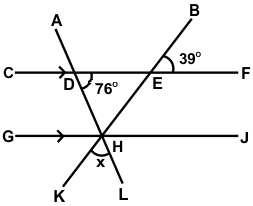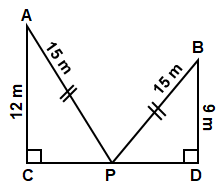# CREST Mathematics Olympiad Class 7 Sample Papers

REGISTER NOW

## Syllabus:

Section 1: Integers, Fractions and Decimals, Exponents and Powers, Algebraic Expressions, Simple Linear Equations, Lines and Angles, Comparing Quantities, The Triangle and its Properties, Symmetry, Congruence of Triangles, Rational Numbers, Perimeter and Area, Data Handling, Visualizing Solid Shapes, Practical Geometry.

Achievers Section: Higher Order Thinking Questions - Syllabus as per Section 1

 Q.1 In an isosceles trapezium PQRS, if line PQ || line RS and ∠QRS = 108⁰, then find the measure of ∠RSP:
 Q.2 Find the value of ∠x:Q.3 Two numbers are in the ratio 17:45. one-third of the smaller is less than One-fifth of the bigger by 15. The smaller number is:
 Q.4 In a certain store, the profit is 320% of the cost. If the cost increases by 25% but the selling price remains constant, then approximately what percentage of the selling price is the profit?
 Q.5 If 2a = 4b = 16c = 256, then what is the value of 2a + 3b + 4c?
 Q.6 The HCF of (x2 - 4) (x + 2) and (x + 2)2 (x3 - 8) is ______.
 Q.7 Find the length of CD:Q.8 If two numbers are in the ratio 2:3 and the product of their HCF and LCM is 33750, then the sum of the numbers is:
 Q.9 The LCM of two numbers is 45 times their HCF. If one of the numbers is 125 and the sum of HCF and LCM is 1150, the other number is:
 Q.10 Ross scored 61 out of 100 in English, 93 out of 100 in Maths and 56 out of 100 in Science. Find out his overall % of marks:

Sample PDF of CREST Mathematics Olympiad for Class 7: